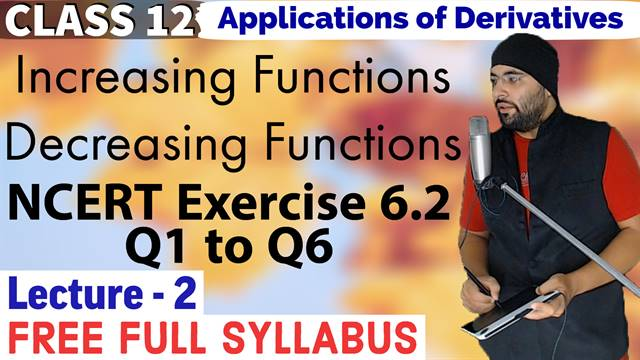# Exercise 6.2 Application of Derivatives Class 12 Maths Chapter 6## Topics/Questions discussed in this video:

In this lecture, I am discussing how to find intervals in which the given function is increasing and decreasing. Also, questions based on this topic from NCERT Exercise 6.2

Questions discussed in this lecture:

 NCERT EXERCISE 6.2 (Increasing and Decreasing Functions)

Question 1. Show that the function given by f (x) = 3x + 17 is increasing on R.

Question 2. Show that the function given by f (x) = e2x is increasing on R.

Question 3. Show that the function given by f (x) = sin x is
(a) increasing in \left( 0, \frac{\pi}{2} \right)
(b) decreasing in \left( \frac{\pi}{2}, \pi \right)
(a) neither increasing nor decreasing in (0, pi)

Question 4. Find the intervals in which the function f given by f(x) = 2x^2 + 3x is
(a) increasing
(b) decreasing

Question 5. Find the intervals in which the function f given by f(x) = 2x^3 - 3x^2 - 36x + 7 is
(a) increasing
(b) decreasing

Question 6. Find the intervals in which the following functions are strictly increasing or decreasing:
(a) x^2 + 2x -5
(b) 10 - 6x - 2x^2
(c) -2x^3 - 9x^2 - 12x + 1
(d) 6 - 9x - x^2